### Home > APCALC > Chapter 8 > Lesson 8.2.3 > Problem8-93

8-93.

Multiple Choice: The graph of $y = f ^\prime(x)$ is shown at right. Which of the graphs below could be the graph of$y = f (x)$?

1.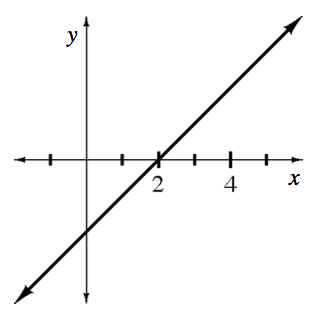1.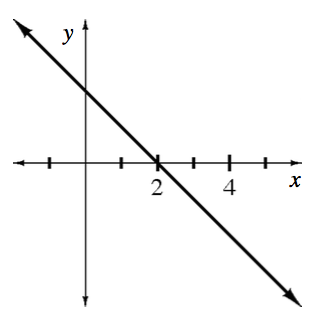1.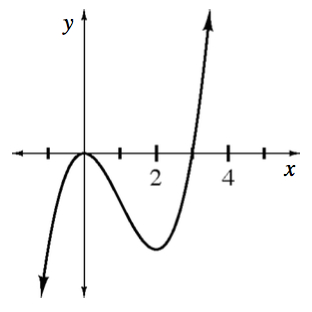1.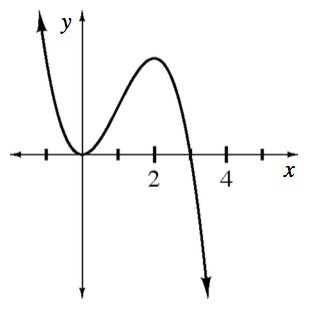1.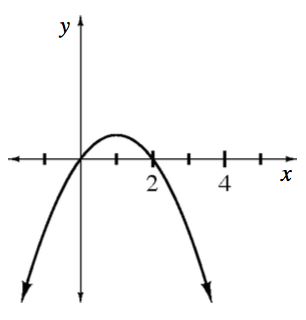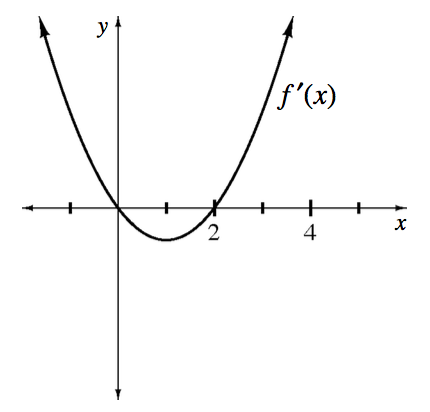$f ^\prime(x)$ appears to be a parabola. What family of functions has a derivative that is a parabola?

$f\left(x\right)$ shows the slopes of $f(x)$. What is significant about the slopes before $x = 0$ and after $x = 2$?